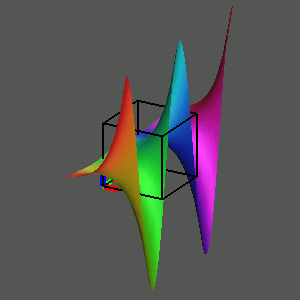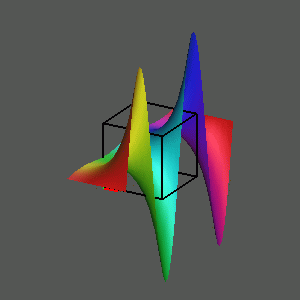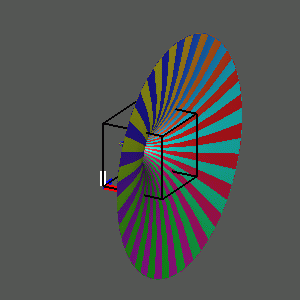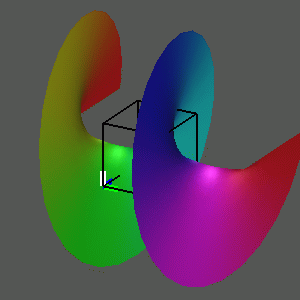# The Complex Exponential and Complex Logarithm

Once we understand how to visualize the graphs of relatively uncomplicated complex functions such at the powers of z, we can use the same techniques to investigate other complex functions such as the complex exponential function w = e^z and its inverse relation, the complex logarithm z = ln(w). In this case, we use a rectangular domain -1<= x <= 1, -2pi <= y <= 2pi. As we rotate this graph in four-space to move from the graph of the real part to the graph of the imaginary part, the surface appears to change phase and wave along.To observe this, play the movie

The inverse relation, the complex logarithm, has a quite complicated Riemann surface, with a real part that is a surface of revolution of the graph of the exponential function in the plane and an imaginary part that is a right helicoid, resembling a circular staircase.To observe this, play the movie

We summarize this investigation of the graph of the complex cube (and its inverse, the complex cube root), by situating the four images at the vertices of a diagram, with arrows between certain vertices. Selecting any vertex plays a movie of a rotation of that object in three- space, and selecting an arrow plays the rotation in four-space from one view to the other.